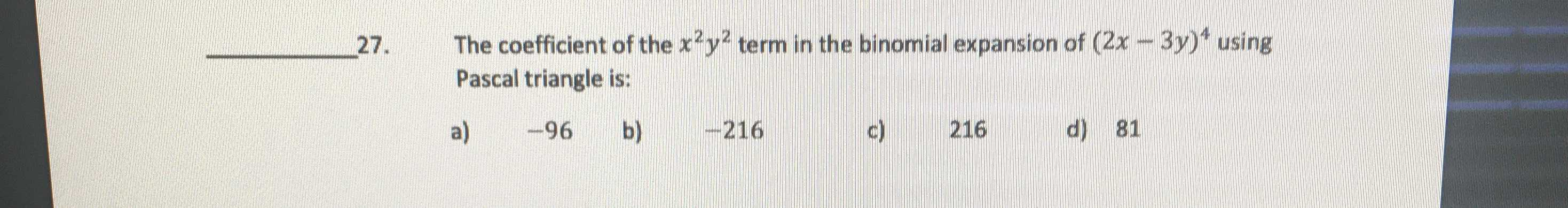### ¿Todavía tienes preguntas de matemáticas?

Pregunte a nuestros tutores expertos
Algebra
Pregunta27. The coefficient of the $$x ^ { 2 } y ^ { 2 }$$ term in the binomial expansion of $$( 2 x - 3 y ) ^ { 4 }$$ using Pascal triangle is: a) $$- 96$$ b) $$- 216$$ c) $$216$$ d) $$81$$